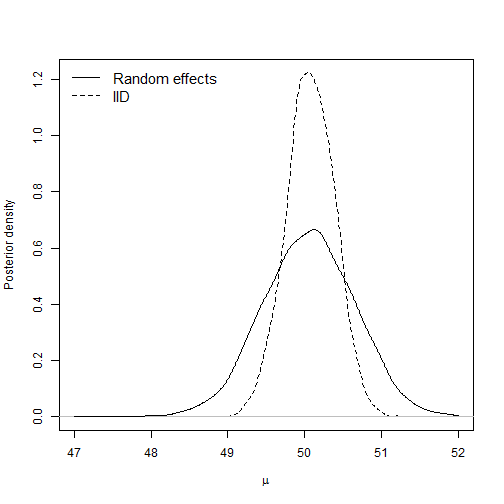# One-way random effects model for the jaw data

### Chapter 4.4: Random effects

Let $$Y_{ij}$$ be the $$j^{th}$$ measurement of jaw bone density for patient $$i$$. The one-way random effects model is

$Y_{ij}|\alpha_i\sim\mbox{Normal}(\alpha_i,\sigma^2)\mbox{ where }\alpha_i\sim\mbox{Normal}(\mu,\tau^2).$

The random effect $$\alpha_i$$ is the true mean for patient $$i$$, and the observations for patient $$i$$ vary around $$\alpha_i$$ with variance $$\sigma^2$$. In this model, the population of patient-specific means is assumed to follow a normal distribution with mean $$\mu$$ and variance $$\tau^2$$. The hyperparameters have uninformative prior $$\mu\sim\mbox{Normal}(0,1000)$$, $$\sigma^2\sim\mbox{InvGamma}(0.1,0.1)$$, and $$\tau^2\sim\mbox{InvGamma}(0.1,0.1)$$.

The objective is to borrow strength across patients to estimate the mean for each patient, $$\alpha_i$$, and to estimate the overall population mean $$\mu$$.

## Load and plot the data

 library(rjags)

m   <- 4
n   <- 20
age <- c(8.0, 8.5, 9.0, 9.5)
Y   <- c(47.8, 48.8, 49.0, 49.7,
46.4, 47.3, 47.7, 48.4,
46.3, 46.8, 47.8, 48.5,
45.1, 45.3, 46.1, 47.2,
47.6, 48.5, 48.9, 49.3,
52.5, 53.2, 53.3, 53.7,
51.2, 53.0, 54.3, 54.5,
49.8, 50.0, 50.3, 52.7,
48.1, 50.8, 52.3, 54.4,
45.0, 47.0, 47.3, 48.3,
51.2, 51.4, 51.6, 51.9,
48.5, 49.2, 53.0, 55.5,
52.1, 52.8, 53.7, 55.0,
48.2, 48.9, 49.3, 49.8,
49.6, 50.4, 51.2, 51.8,
50.7, 51.7, 52.7, 53.3,
47.2, 47.7, 48.4, 49.5,
53.3, 54.6, 55.1, 55.3,
46.2, 47.5, 48.1, 48.4,
46.3, 47.6, 51.3, 51.8)

Y <- matrix(Y,20,4,byrow=TRUE)

plot(row(Y),Y,xlab="Patient",ylab="Bone density",pch=19)
lines(rowMeans(Y))
legend("topleft",c("Observations","Sample mean"),lty=c(NA,1),pch=c(19,NA),bty="n")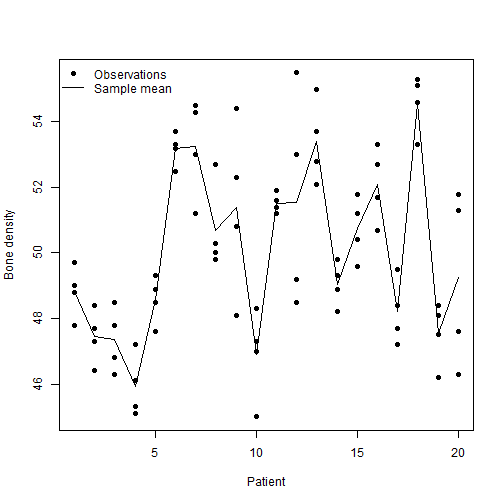## Put the data in JAGS format

 data     <- list(Y=Y,n=n,m=m)
burn     <- 10000
n.iter   <- 20000
thin     <- 20
n.chains <- 2


## (1) Fit the one-way random effects model with Gamma priors

 model_string <- textConnection("model{

# Likelihood
for(i in 1:n){for(j in 1:m){
Y[i,j] ~ dnorm(alpha[i],taue)
}}

# Random effects
for(i in 1:n){alpha[i] ~ dnorm(mu,taua)}

# Priors
mu   ~ dnorm(0,0.0001)
taue ~ dgamma(0.1,0.1)
taua ~ dgamma(0.1,0.1)
}")

params   <- c("mu","alpha","taue","taua")
model    <- jags.model(model_string, data = data,
n.chains=n.chains, quiet=TRUE)
update(model, burn, progress.bar="none")
samples1 <- coda.samples(model, variable.names=params, thin=thin,
n.iter=n.iter, progress.bar="none")

samples1 <- rbind(samples1[],samples1[])
alpha    <- samples1[,1:n]
mu       <- samples1[,n+1]
sigma2   <- 1/samples1[,n+2:3]
r        <- sigma2[,2]/rowSums(sigma2)
hist(r,breaks=50,prob=TRUE,main="",xlab="Proportion of variance explained by the random effect")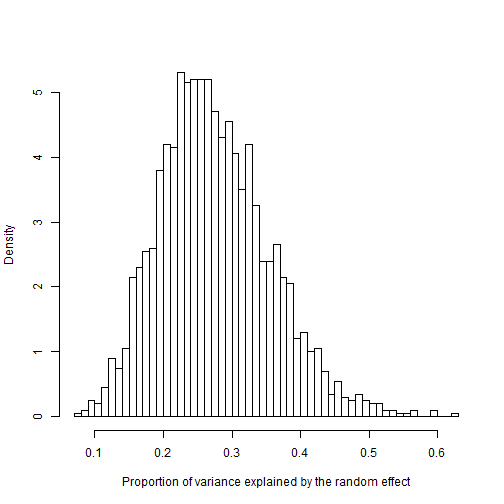## Random-effect estimates

The plots the posterior of each subject's random effect, $$\alpha_i$$, as a boxplot. The data $$Y_{ij}$$ are overlain as points.

 boxplot(alpha~col(alpha),ylim=range(Y),xlab="Patient",ylab="Bone density",outline=FALSE)
points(row(Y),Y,pch=19)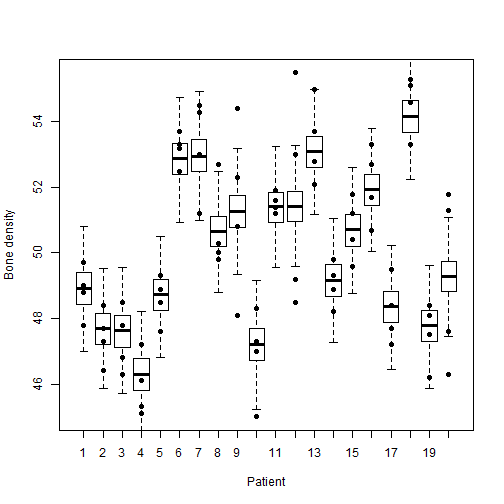## (2) Fit the one-way random effects model with half-Cauchy priors

 model_string_HC <- textConnection("model{

# Likelihood
for(i in 1:n){for(j in 1:m){
Y[i,j] ~ dnorm(alpha[i],taue)
}}

# Random effects
for(i in 1:n){alpha[i] ~ dnorm(mu,taua)}

# Priors
mu      ~ dnorm(0,0.0001)
taue    <- pow(sigma1,-2)

taua    <- pow(sigma2,-2)

sigma1   ~ dt(0, 1, 1)T(0,)
sigma2   ~ dt(0, 1, 1)T(0,)

}")

model    <- jags.model(model_string_HC, data = data,
n.chains=n.chains, quiet=TRUE)
update(model, burn, progress.bar="none")
samplesHC <- coda.samples(model, variable.names=params, thin=thin,
n.iter=n.iter, progress.bar="none")

samplesHC <- rbind(samplesHC[],samplesHC[])
sigma2HC <- 1/samplesHC[,n+2:3]


## Prior sensitivity

The summaries below compare the posterior distribution of the standard deviation using InvGamma versus half-Cauchy priors. For these data the results are similar for the two priors.

  apply(sqrt(sigma2),2,quantile,c(0.5,0.025,0.975))   # InvGamma prior

##           taua     taue
## 50%   2.421446 1.467340
## 2.5%  1.757569 1.243607
## 97.5% 3.512755 1.778204

  apply(sqrt(sigma2HC),2,quantile,c(0.5,0.025,0.975)) # Half-Cauchy prior

##           taua     taue
## 50%   2.374449 1.465342
## 2.5%  1.724867 1.242088
## 97.5% 3.456168 1.770958

  plot(density(sigma2[,1]),xlab="Sigma",ylab="Posterior",main="Error SD")
lines(density(sigma2HC[,1]),col=2)
legend("topright",c("InvGamma","Half-Cauchy"),lty=1,col=1:2,bty="n")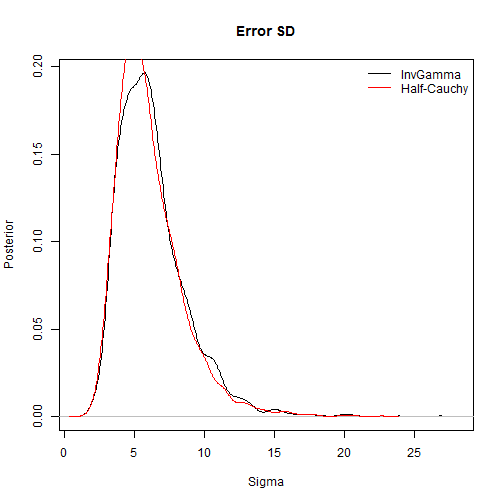plot(density(sigma2[,2]),xlab="Sigma",ylab="Posterior",main="Random effect SD")
lines(density(sigma2HC[,2]),col=2)
legend("topright",c("InvGamma","Half-Cauchy"),lty=1,col=1:2,bty="n")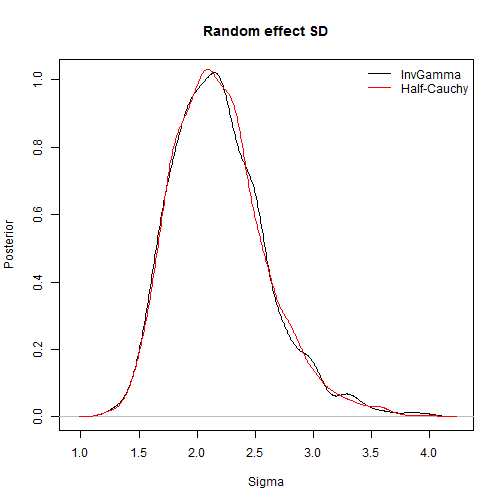## Comparison with naive model

In addition to estimating random effects, random-effect models are useful to account for correlation between observations to obtain valid uncertainty estimates for model parameters. For example, say our objective is to estimate $$\mu$$. We could do this assuming all $$n*m=80$$ observations are independent. But because we ignore dependence between repeated measurements for each subject, this inference is questionable.

 model_string0 <- textConnection("model{
# Likelihood
for(i in 1:n){for(j in 1:m){
Y[i,j] ~ dnorm(mu,taue)
}}

# Priors
mu ~ dnorm(0,0.0001)
taue ~ dgamma(0.1,0.1)
}")

model0   <- jags.model(model_string0,data = data,
n.chains=2, quiet=TRUE)
update(model0, burn, progress.bar="none")
samples0 <- coda.samples(model0, variable.names=c("mu"),
n.iter=n.iter, thin=thin, progress.bar="none")
mu_naive <- c(samples0[],samples0[])


The posterior of $$\mu$$ has smaller variance under the naive model because it does not account for dependence.

 d1 <- density(mu,from=47,to=52)
d0 <- density(mu_naive,from=47,to=52)
quantile(mu,c(0.025,0.975))

##     2.5%    97.5%
## 48.88611 51.20441

 quantile(mu_naive,c(0.025,0.975))

##     2.5%    97.5%
## 49.45833 50.67698

 var(mu)/var(mu_naive)

##  3.673447

 plot(d0,type="l",lty=2,xlab=expression(mu),ylab="Posterior density",main="")
lines(d1,lty=1)

legend("topleft",c("Random effects","IID"),lty=1:2,bty="n",cex=1.25)